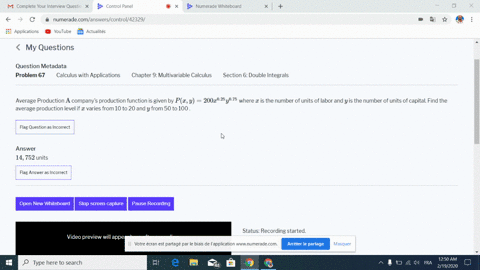🎉 The Study-to-Win Winning Ticket number has been announced! Go to your Tickets dashboard to see if you won! 🎉View Winning Ticket### Average Production $\mathrm{A}$ company's product…

18:13Georgia Southern University
Problem 66

# Average cost A restaurant's cost function is approximated by$C(x, y)=\frac{1}{4} x^{2}+4 x+2 y^{2}+y+10$dollars, where $x$ represents the cost of labor per hour and $y$ represents the average cost of materials per dish. Find the average cost of the restaurant per hour if the cost of labor per hour in therestaurant is between $\$ 30$and$\$60,$ and the cost of material perdish is between $\$ 50$and$\$80 .$

## Discussion

You must be signed in to discuss.

## Video Transcript

No transcript available